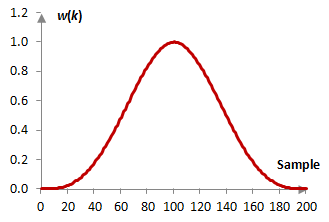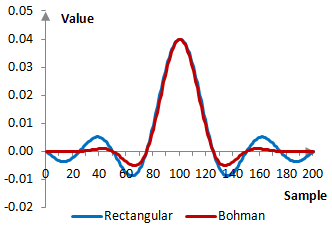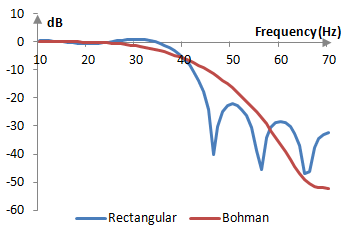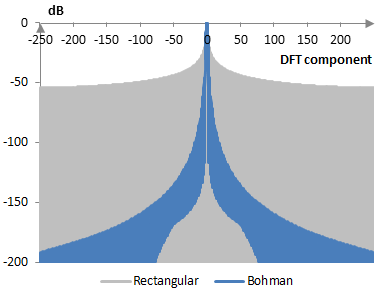# Bohman window

The Bohman window coefficients are given by the following formula

$$w(k)=(1-|\frac{k}{M}-1|)\,\cos(\pi |\frac{k}{M}-1|)+\frac{1}{\pi}\,\sin(\pi |\frac{k}{M}-1|)$$

where N is the length of the filter, M = (N – 1) / 2, and k = 0, 1, …, N – 1.

The Bohman window is the convolution of the sine window with itself.

## An example Bohman window

Take a finite impulse response (FIR) low pass filter of length N = 201. The following is the Bohman window.Given a sampling frequency of 2000 Hz and a filter cutoff frequency of 40 Hz, the impulse response of the filter with a rectangular window (with no window) and with the Bohman window is as follows.The magnitude response of the same filter is shown on the graph below.## Measures for the Bohman window

The following is a plot of the discrete Fourier transform of the Bohman window against the discrete Fourier transform of the rectangular window.The measures of the Bohman window are as follows.

 Coherent gain 0.40 Equivalent noise bandwidth 1.79 Processing gain -2.53 dB Scalloping loss -1.02 dB Worst case processing loss -3.55 dB Highest sidelobe level -46.0 dB Sidelobe falloff -21.4 dB / octave, -71.0 dB / decade Main lobe is -3 dB 1.70 bins Main lobe is -6 dB 2.38 bins Overlap correlation at 50% overlap 0.073 Amplitude flatness at 50% overlap 0.637 Overlap correlation at 75% overlap 0.544 Amplitude flatness at 75% overlap 0.982

Window

### Filtered HTML

• Freelinking helps you easily create HTML links. Links take the form of [[indicator:target|Title]]. By default (no indicator): Click to view a local node.